Author: Oscar Cronquist Article last updated on February 08, 2023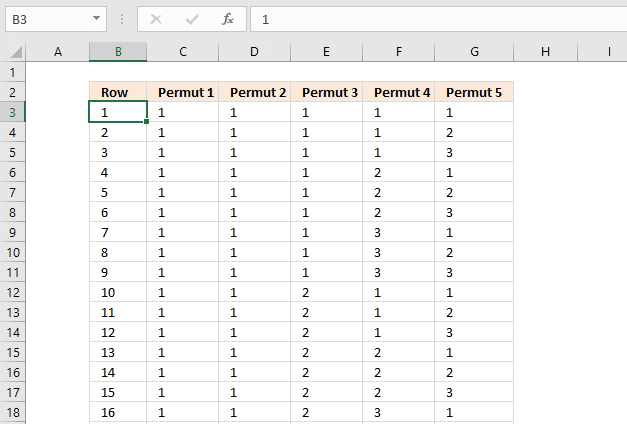This article demonstrates a macro that lets you create a list of permutations, repetitions are allowed, based on a number of comma-delimited strings and the number of items per row.

The image above shows a list created by the macro based on numbers 1, 2, and 3 and five items per row.

Is there a way where I can predict all possible outcomes in Excel in the below example?

Total games are 13 (ABCDEFGHIJKLM). Possible outcomes are win(home team), draw or win(Away team) represented by 1, X or 2 respectively. Outcomes should be in the below formats:

111111111111X
11111111111XX
2222222X22221
222222222222X

## 2. VBA code

The following macro lets you enter text strings separated by a comma and the number of games.

```Sub ListPermut()
'This macro creates a list of all permutations
'Get digital Help - https://www.get-digital-help.com/

'Define variables
Dim ws As Worksheet, Ans As String, ans1() As String, digits As Integer
Dim num As Integer, p As Long, i As Long, t As Long
Dim rng() As Long, c As Long, rng1() As String

'Insert a new sheet

Ans = InputBox("Type strings separated with a comma:")
digits = InputBox("How many strings?")

'Split text strings to an array
ans1 = Split(Ans, ",")

'Count values in aray
num = UBound(ans1) + 1

'Calculate number of permutations
p = num ^ digits

'Redimension arrays
ReDim rng(1 To digits)
ReDim rng1(1 To digits)

'Save 1 to all values in first row of array
For c = 1 To digits
rng(c) = 1
Next c

i = 0

'Don't show the result until finished
Application.ScreenUpdating = False

'Repeat until all permutations have been created
Do Until (i + t) = (p - 1)
'Use text strings instead of numbers
For c = LBound(rng1) To UBound(rng1)
rng1(c) = ans1(rng(c) - 1)
Next c
'Transfer values from array to worksheet
ws.Range("A1").Resize(, digits).Offset(i) = rng1

'Build next row of permutations
For c = digits To 1 Step -1
If c = digits Then
rng(c) = rng(c) + 1
ElseIf rng(c) = 0 Then
rng(c) = rng(c - 1)
End If
If rng(c) = num + 1 Then
rng(c) = 1
rng(c - 1) = rng(c - 1) + 1
End If
Next c

i = i + 1

'Insert a new sheet if rows exceed 999 999
If i = 1000000 Then
t = t + 1000000
i = 0
End If

Loop
'Use text strings instead of numbers
For c = LBound(rng1) To UBound(rng1)
rng1(c) = ans1(rng(c) - 1)
Next c
'Transfer values from array to worksheet
ws.Range("A1").Resize(, digits).Offset(i) = rng1
'Show output
Application.ScreenUpdating = True

End Sub
```

## 3. Where to copy code?

1. Press Alt-F11 to open visual basic editor
2. Press with left mouse button on Module on the Insert menu
3. Copy and paste code above to the code module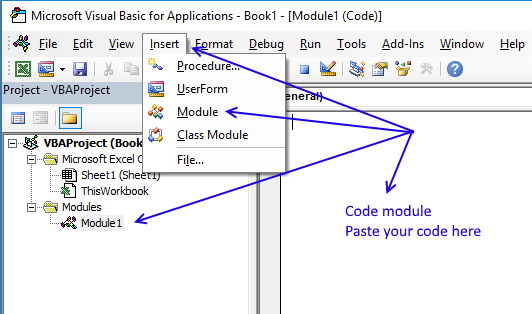4. Exit visual basic editor (Alt+Q)
Note, save workbook with file extension *.xlsm (macro-enabled) to keep code attached to workbook.

## 4. How to run the macro?

1. Press Alt+F8 to open the macro dialog box
2. Press with left mouse button on ListPermut macro
3. Press with left mouse button on Run.

## 5. How to use the macro?

Enter your text strings using a comma as a text delimiting character.Press with left mouse button on OK button.

Enter number of games.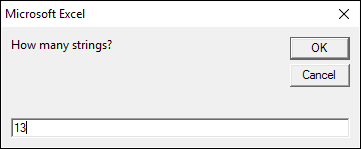Press with left mouse button on OK button.

Excel processes the data for a while and then creates two sheets with a total of 1594323 permutations.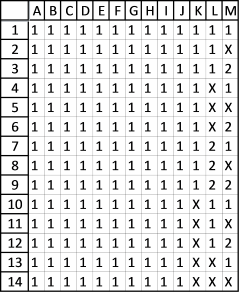The picture shows 14 out of 1594323 permutations.

## 6. List permutations with repetition and how many to choose from (Excel 365 formula)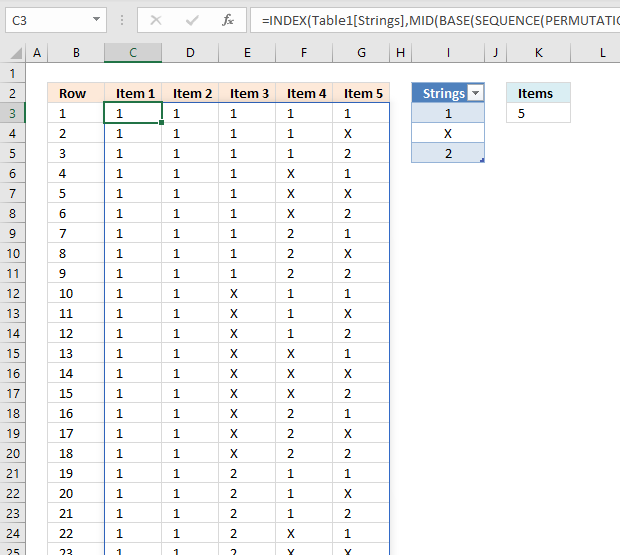The Excel 365 formula in cell C3 spills to other adjacent cells automatically based on the values in the Excel Table (cell range I3:I5) and the number entered in cell K3.

You can add and delete strings in cell range I3:I5, the only limit is the worksheet row limit of 1 048 576. For example, 3 strings and 13 items return 1 594 323 rows and won't fit a worksheet.

You have to move the Excel Table and the value in cell K3 if you have more than 6 items, the Excel 365 formula returns a #SPILL! error if adjacent cells are populated.

Excel 365 dynamic array formula in cell C3:

=INDEX(Table1[Strings], MID(BASE(SEQUENCE(PERMUTATIONA(COUNTA(Table1[Strings]),\$K\$3))-1, COUNTA(Table1[Strings]), \$K\$3), SEQUENCE(, \$K\$3), 1)+1)

Excel 365 formula in cell B3:

=SEQUENCE(PERMUTATIONA(COUNTA(Table1[Strings]), K3))

### Get the Excel fileListPermutationsv3.xlsm